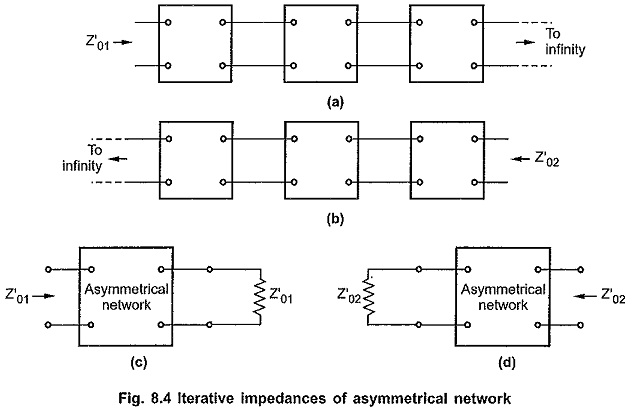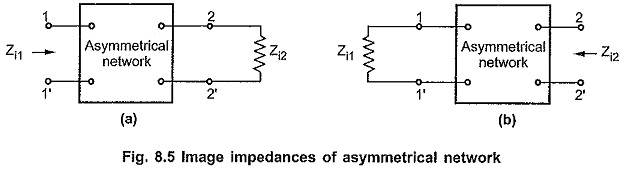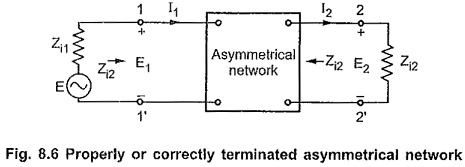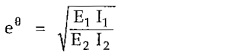## Asymmetrical Network in Network Analysis:

An asymmetrical network has following electrical properties,

• Iterative impedance
• Image impedance
• Image transfer constant

### Iterative impedance (Z′0):

Consider that infinite asymmetrical networks having identical electrical properties are connected in cascade as shown in the Fig. 8.4 (a) and (b).The iterative impedance is the impedance measured at one pair of terminals of the network in the chain of infinite networks as shown in the Fig. 8.4 (a) and (b). This is the impedance measured at any pair of terminals of the network when other pair of terminals is terminated in the impedance of the same value as shown in the Fig. 8.4 (c) and (d).

The iterative impedances for any asymmetrical network are of different values when measured at different ports of the network. The iterative impedances are represented by Z′01 and Z’02 respectively at port 1 and port 2.

### Image impedances (Zi):

Similar to the iterative impedances, the image impedances are also of different values at different ports. Let the image impedances be denoted by Zi1 and Zi2. Consider that the asymmetrical network is terminated with image impedance of port 2 Zi2 at its output pair of terminals then the impedance measured at its input pair of terminals will be image impedance of port 1 i.e. Zi1. Similarly if port one is terminated in the image impedance of port 1 i.e. Zi1 then the impedance measured at port two will be the image impedance of port 2 i.e. Zi2. These conditions are illustrated by the Fig. 8.5 (a) and (b).When an asymmetrical network is terminated in image impedances at both the ports the network is called correctly terminated asymmetrical network as shown in the Fig. 8.6.### Image transfer constant (eθ):

When an asymmetrical network is terminated in its image impedances at both the ports as shown in the Fig. 8.6, then the ratio of currents I1/I2 will be different from the E1/E2.

Hence image transfer constant θ is defined asThe real part of image transfer constant is called image attenuation constant; while the imaginary part is called image phase constant.

After detailed discussion of various properties of symmetrical and asymmetrical networks let us study the properties of some of the important symmetrical and asymmetrical networks including symmetrical T, π, lattice networks and asymmetrical networks such as half sections, L sections.

Scroll to Top